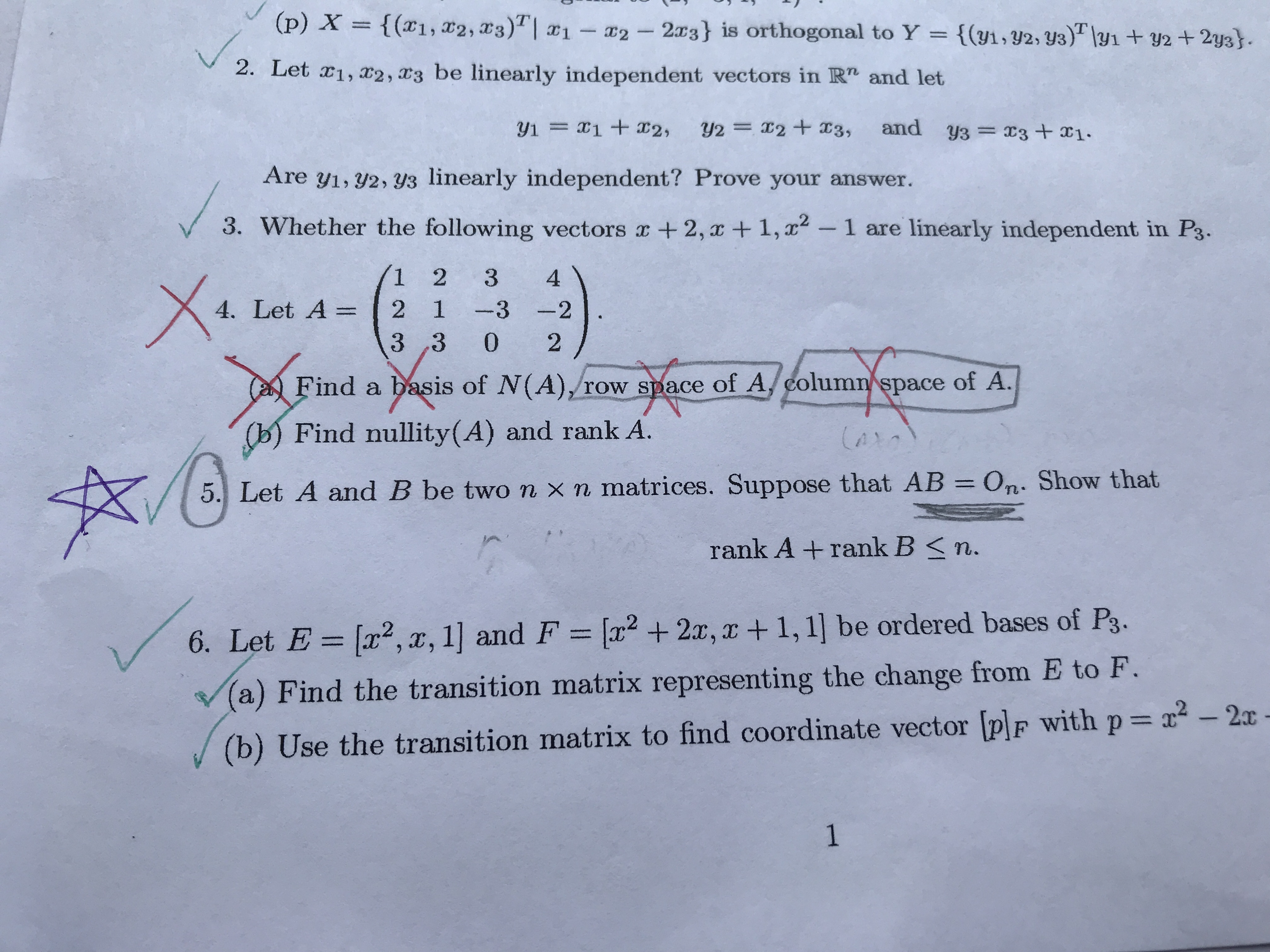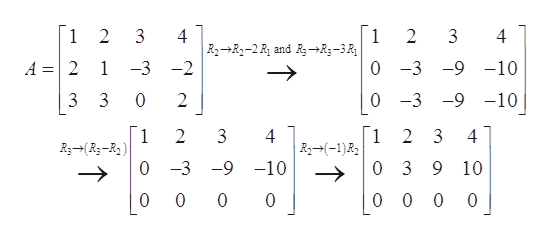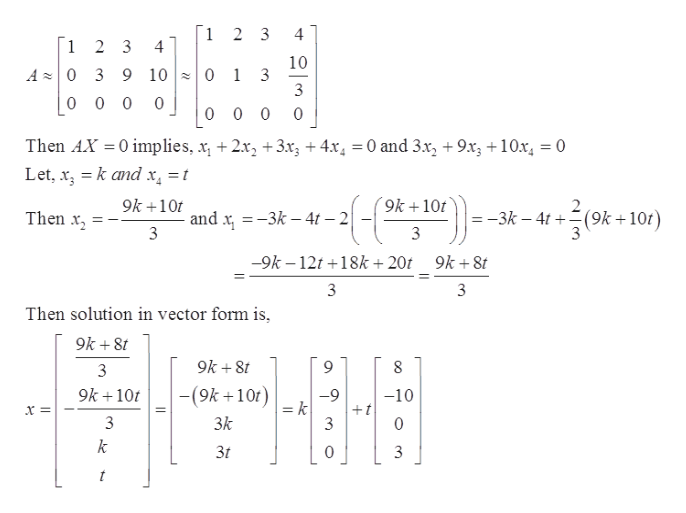# (p) X{(x1, 22, 3)| x1- 2-23} is orthogonal to Y = {(y1,y2, y3) 1y1+y2+2y3T2. Let 1, 2, 3 be linearly independent vectors in R" and lety1 x1 ax2,Y2 = x2 + X3,and y3=3+x1Are y1, y2, 3y3 linearly independent? Prove your answer.3. Whether the following vectors x + 2,x +1,1 are linearly independent in P3.1 2 342 1-3 23 3 04. Let A =2Find a basis of N(A),/row space of A/ column space of A.(b) Find nullity (A) and rank A.5. Let A and B be two n xn matrices. Suppose that AB On. Show thatrank A + rank B

Question
5 views

4ahelp_outlineImage Transcriptionclose(p) X {(x1, 22, 3)| x1- 2-23} is orthogonal to Y = {(y1,y2, y3) 1y1+y2+2y3 T 2. Let 1, 2, 3 be linearly independent vectors in R" and let y1 x1 ax2, Y2 = x2 + X3, and y3=3+x1 Are y1, y2, 3y3 linearly independent? Prove your answer. 3. Whether the following vectors x + 2,x +1, 1 are linearly independent in P3. 1 2 3 4 2 1-3 2 3 3 0 4. Let A = 2 Find a basis of N(A),/row space of A/ column space of A. (b) Find nullity (A) and rank A. 5. Let A and B be two n xn matrices. Suppose that AB On. Show that rank A + rank B
check_circle

star
star
star
star
star
1 Rating
Step 1

Consider the given matrix A as,

Step 2

On solving matrix A to reduce in row-echelon form,help_outlineImage Transcriptionclose1 3 4 1 2 3 4 2 0 -3 A =2 -9 -10 1 -3 -2 0 -3 -9 -10 3 0 2 Г1 2 3 1 R3-R-R 2 4 R2)R 4 о -3 -9 -10 0 3 9 10 0 0 0 0 0 0 0 fullscreen
Step 3

General solution of...help_outlineImage Transcriptionclose1 2 3 4 1 2 3 4 10 A 0 3 9 10 0 1 3 0 0 0 0 0 0 0 Then AX 0 implies, x 2x, +3x3 +4x4 = 0 and 3x2 +9x3 +10x = 0 Let, x kand x = t 9k10 9k+10t 2 (9k +10t) Then x and x 3k-4t 2 3 -3k -4t 9k-12t+18k+20t 9k+8t 3 Then solution in vector form is, 9k+8t 9k+8t 8 3 9k+ 10 -(9k 10t) -10 -9 = k +t 3 3k 3 0 k 3t 0 t fullscreen

### Want to see the full answer?

See Solution

#### Want to see this answer and more?

Solutions are written by subject experts who are available 24/7. Questions are typically answered within 1 hour.*

See Solution
*Response times may vary by subject and question.
Tagged in

### Math